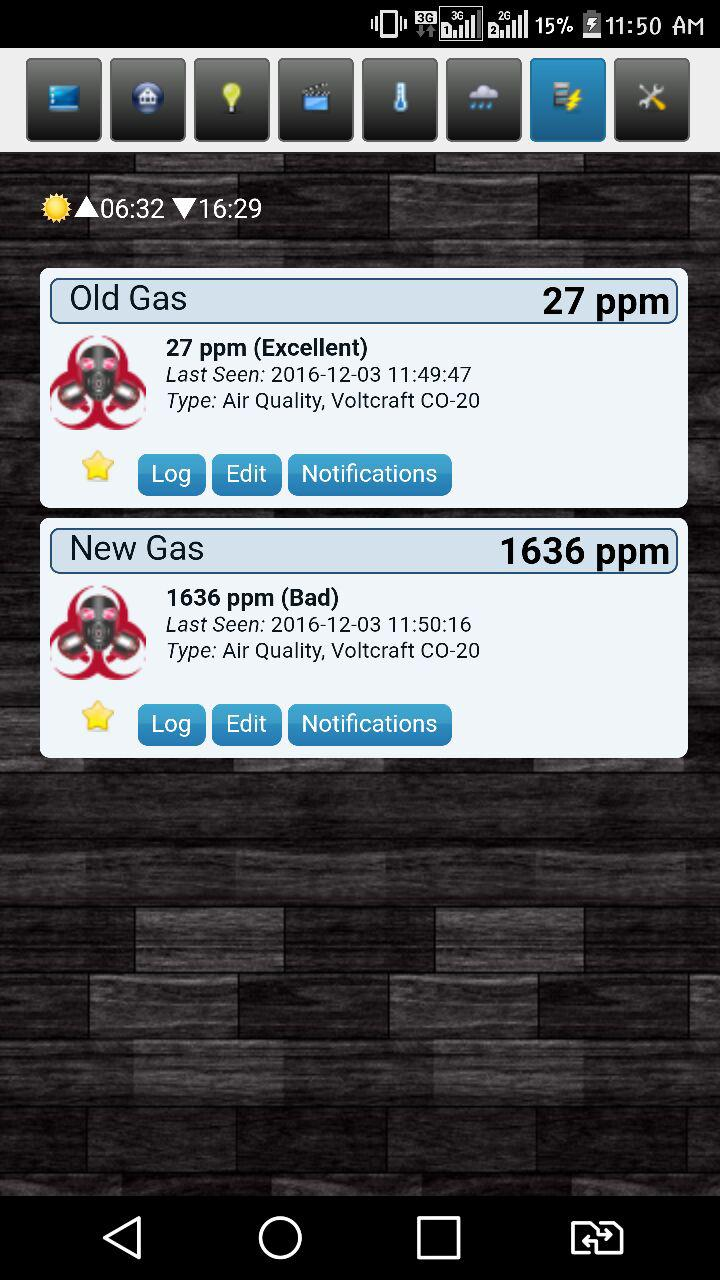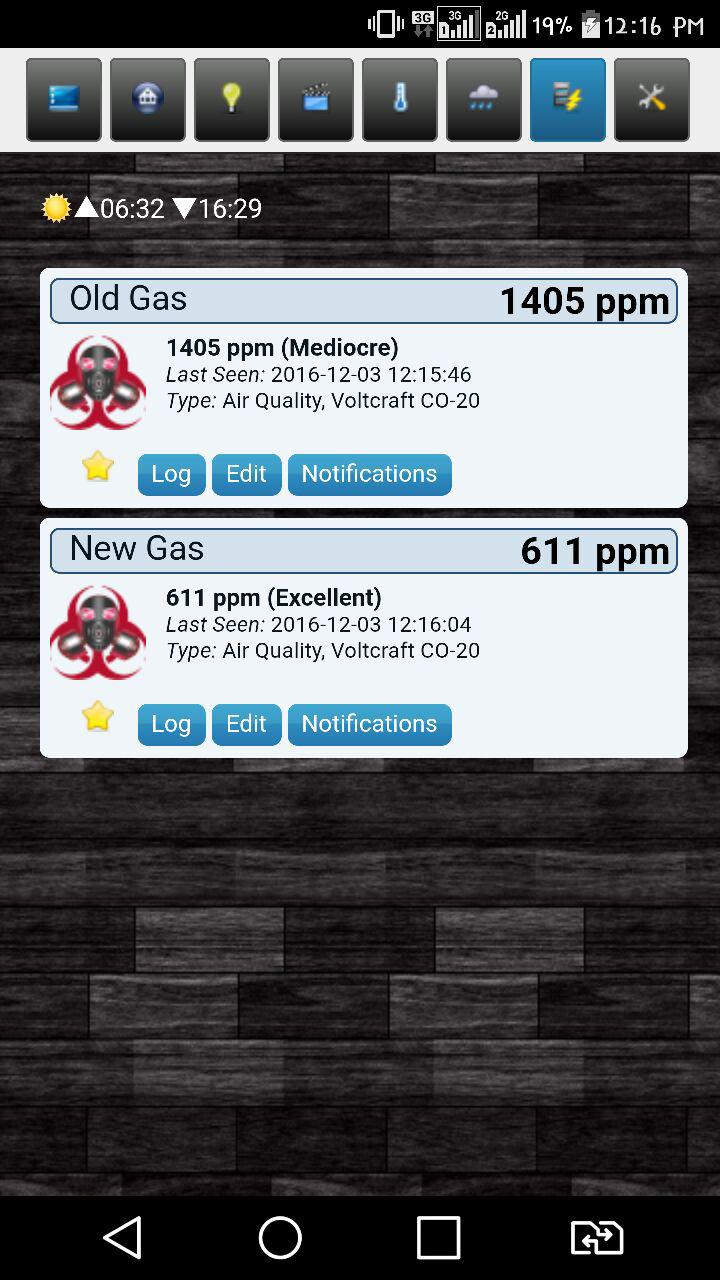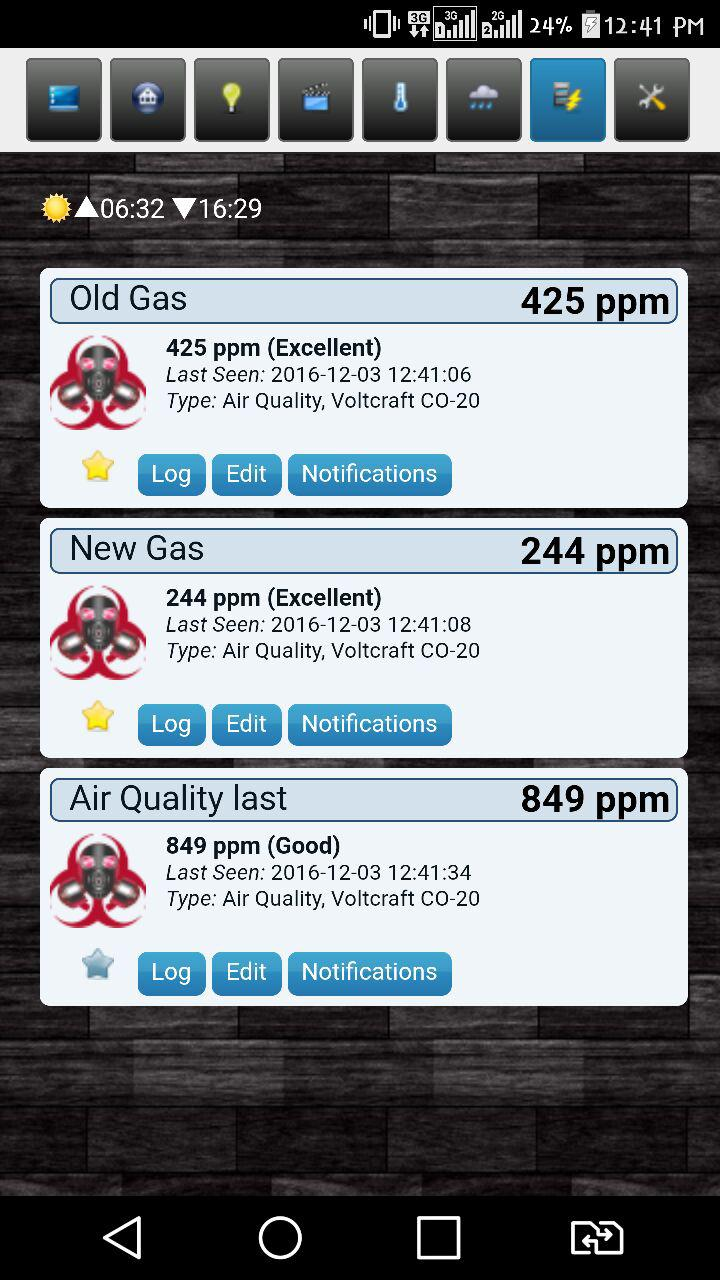• hi guys
i have 2 problem with gas sensor

``````#define MY_DEBUG
#define MY_RF24_CHANNEL 0
#define MY_NODE_ID 4
#define MY_REPEATER_FEATURE

#include <MySensors.h>
#include <avr/wdt.h>

unsigned long time_m;
unsigned long a , b , c;

#define     CHILD_ID_MQ                   0
/************************Hardware Related Macros************************************/
#define     MQ_SENSOR_ANALOG_PIN         (0)  //define which analog input channel you are going to use
#define         RL_VALUE                     (5)     //define the load resistance on the board, in kilo ohms
#define         RO_CLEAN_AIR_FACTOR          (9.83)  //RO_CLEAR_AIR_FACTOR=(Sensor resistance in clean air)/RO,
//which is derived from the chart in datasheet
/***********************Software Related Macros************************************/
#define         CALIBARAION_SAMPLE_TIMES     (50)    //define how many samples you are going to take in the calibration phase
#define         CALIBRATION_SAMPLE_INTERVAL  (500)   //define the time interal(in milisecond) between each samples in the
//cablibration phase
#define         READ_SAMPLE_INTERVAL         (50)    //define how many samples you are going to take in normal operation
#define         READ_SAMPLE_TIMES            (5)     //define the time interal(in milisecond) between each samples in
//normal operation
/**********************Application Related Macros**********************************/
#define         GAS_LPG                      (0)
#define         GAS_CO                       (1)
#define         GAS_SMOKE                    (2)
/*****************************Globals***********************************************/
//unsigned long SLEEP_TIME = 30000; // Sleep time between reads (in milliseconds)
//VARIABLES
float Ro = 10000.0;    // this has to be tuned 10K Ohm
int val = 0;           // variable to store the value coming from the sensor
float valMQ = 0.0;
float lastMQ = 0.0;
float           LPGCurve  =  {2.3, 0.21, -0.47}; //two points are taken from the curve.
//with these two points, a line is formed which is "approximately equivalent"
//to the original curve.
//data format:{ x, y, slope}; point1: (lg200, 0.21), point2: (lg10000, -0.59)
float           COCurve  =  {2.3, 0.72, -0.34};  //two points are taken from the curve.
//with these two points, a line is formed which is "approximately equivalent"
//to the original curve.
//data format:{ x, y, slope}; point1: (lg200, 0.72), point2: (lg10000,  0.15)
float           SmokeCurve = {2.3, 0.53, -0.44}; //two points are taken from the curve.
//with these two points, a line is formed which is "approximately equivalent"
//to the original curve.
//data format:{ x, y, slope}; point1: (lg200, 0.53), point2:(lg10000,-0.22)

MyMessage msg(CHILD_ID_MQ, V_LEVEL);

void setup()
{
Ro = MQCalibration(MQ_SENSOR_ANALOG_PIN);         //Calibrating the sensor. Please make sure the sensor is in clean air
wdt_enable(WDTO_4S);
}

void presentation() {
// Send the sketch version information to the gateway and Controller
sendSketchInfo("R_M Gas", "1.0");

// Register all sensors to gateway (they will be created as child devices)
present(CHILD_ID_MQ, S_AIR_QUALITY);
}

void loop()
{
time_m = millis();
a = time_m / 1000;
b = a % 40;
c = a % 20;
if (b == 0) {
uint16_t valMQ = MQGetGasPercentage(MQRead(MQ_SENSOR_ANALOG_PIN) / Ro, GAS_CO);
Serial.println(val);

Serial.print("LPG:");
Serial.print( "ppm" );
Serial.print("    ");
Serial.print("CO:");
Serial.print( "ppm" );
Serial.print("    ");
Serial.print("SMOKE:");
Serial.print( "ppm" );
Serial.print("\n");
if (valMQ != lastMQ) {
send(msg.set((int16_t)ceil(valMQ)));
lastMQ = ceil(valMQ);
}}

if (c == 0) {
send(msg.set((int16_t)ceil(lastMQ)));
}
wait(1000);
wdt_reset();
// wait(SLEEP_TIME); //sleep for: sleepTime

}

/****************** MQResistanceCalculation ****************************************
Output:  the calculated sensor resistance
Remarks: The sensor and the load resistor forms a voltage divider. Given the voltage
across the load resistor and its resistance, the resistance of the sensor
could be derived.
************************************************************************************/
{
}

/***************************** MQCalibration ****************************************
Input:   mq_pin - analog channel
Output:  Ro of the sensor
Remarks: This function assumes that the sensor is in clean air. It use
MQResistanceCalculation to calculates the sensor resistance in clean air
and then divides it with RO_CLEAN_AIR_FACTOR. RO_CLEAN_AIR_FACTOR is about
10, which differs slightly between different sensors.
************************************************************************************/
float MQCalibration(int mq_pin)
{
int i;
float val = 0;

for (i = 0; i < CALIBARAION_SAMPLE_TIMES; i++) {      //take multiple samples
delay(CALIBRATION_SAMPLE_INTERVAL);
}
val = val / CALIBARAION_SAMPLE_TIMES;                 //calculate the average value

val = val / RO_CLEAN_AIR_FACTOR;                      //divided by RO_CLEAN_AIR_FACTOR yields the Ro
//according to the chart in the datasheet

return val;
}
Input:   mq_pin - analog channel
Output:  Rs of the sensor
Remarks: This function use MQResistanceCalculation to caculate the sensor resistenc (Rs).
The Rs changes as the sensor is in the different consentration of the target
gas. The sample times and the time interval between samples could be configured
by changing the definition of the macros.
************************************************************************************/
{
int i;
float rs = 0;

for (i = 0; i < READ_SAMPLE_TIMES; i++) {
}

return rs;
}

/*****************************  MQGetGasPercentage **********************************
Input:   rs_ro_ratio - Rs divided by Ro
gas_id      - target gas type
Output:  ppm of the target gas
Remarks: This function passes different curves to the MQGetPercentage function which
calculates the ppm (parts per million) of the target gas.
************************************************************************************/
int MQGetGasPercentage(float rs_ro_ratio, int gas_id)
{
if ( gas_id == GAS_LPG ) {
return MQGetPercentage(rs_ro_ratio, LPGCurve);
} else if ( gas_id == GAS_CO ) {
return MQGetPercentage(rs_ro_ratio, COCurve);
} else if ( gas_id == GAS_SMOKE ) {
return MQGetPercentage(rs_ro_ratio, SmokeCurve);
}

return 0;
}

/*****************************  MQGetPercentage **********************************
Input:   rs_ro_ratio - Rs divided by Ro
pcurve      - pointer to the curve of the target gas
Output:  ppm of the target gas
Remarks: By using the slope and a point of the line. The x(logarithmic value of ppm)
of the line could be derived if y(rs_ro_ratio) is provided. As it is a
logarithmic coordinate, power of 10 is used to convert the result to non-logarithmic
value.
************************************************************************************/
int  MQGetPercentage(float rs_ro_ratio, float *pcurve)
{
return (pow(10, ( ((log(rs_ro_ratio) - pcurve) / pcurve) + pcurve)));
}
``````

in this sketch just report 0 value :

``````0 MCO:BGN:INIT REPEATER,CP=RNNRA--,VER=2.0.1-beta
4 TSM:INIT
11 TSM:INIT:TSP OK
12 TSM:INIT:STATID=122
14 TSF:SID:OK,ID=122
16 TSM:FPAR
53 TSF:MSG:SEND,122-122-255-255,s=255,c=3,t=7,pt=0,l=0,sg=0,ft=0,st=OK:
321 TSF:MSG:FPAR OK,ID=0,D=1
2060 TSM:FPAR:OK
2061 TSM:ID
2062 TSM:ID:OK
2064 TSM:UPL
2067 TSF:MSG:SEND,122-122-0-0,s=255,c=3,t=24,pt=1,l=1,sg=0,ft=0,st=OK:1
2082 TSF:MSG:PONG RECV,HP=1
2084 TSM:UPL:OK
2089 TSF:MSG:SEND,122-122-0-0,s=255,c=3,t=15,pt=6,l=2,sg=0,ft=0,st=OK:0100
2107 TSF:MSG:SEND,122-122-0-0,s=255,c=0,t=18,pt=0,l=10,sg=0,ft=0,st=OK:2.0.1-beta
2116 TSF:MSG:SEND,122-122-0-0,s=255,c=3,t=6,pt=1,l=1,sg=0,ft=0,st=OK:0
2509 TSF:MSG:SEND,122-122-0-0,s=255,c=3,t=11,pt=0,l=7,sg=0,ft=0,st=OK:R_M Gas
2519 TSF:MSG:SEND,122-122-0-0,s=255,c=3,t=12,pt=0,l=3,sg=0,ft=0,st=OK:1.0
2528 TSF:MSG:SEND,122-122-0-0,s=0,c=0,t=22,pt=0,l=0,sg=0,ft=0,st=OK:
2534 MCO:REG:REQ
2537 TSF:MSG:SEND,122-122-0-0,s=255,c=3,t=26,pt=1,l=1,sg=0,ft=0,st=OK:2
2549 MCO:PIM:NODE REG=1
2551 MCO:BGN:STP
27561 MCO:BGN:INIT OK,ID=122,PAR=0,DIS=1,REG=1
27572 !TSF:MSG:FPAR INACTIVE
0
LPG:0ppm    CO:0ppm    SMOKE:0ppm
41583 TSF:MSG:SEND,122-122-0-0,s=0,c=1,t=37,pt=2,l=2,sg=0,ft=0,st=OK:0
60591 TSF:MSG:SEND,122-122-0-0,s=0,c=1,t=37,pt=2,l=2,sg=0,ft=0,st=OK:0
71488 TSF:MSG:BC
71489 TSF:MSG:FPAR REQ,ID=8
71492 TSF:PNG:SEND,TO=0
71496 TSF:MSG:SEND,122-122-0-0,s=255,c=3,t=24,pt=1,l=1,sg=0,ft=0,st=OK:1
71512 TSF:MSG:PONG RECV,HP=1
71515 TSF:CKU:OK
71516 TSF:MSG:GWL OK
72384 TSF:MSG:SEND,122-122-8-8,s=255,c=3,t=8,pt=1,l=1,sg=0,ft=0,st=OK:1
0
LPG:0ppm    CO:0ppm    SMOKE:0ppm
81399 TSF:MSG:SEND,122-122-0-0,s=0,c=1,t=37,pt=2,l=2,sg=0,ft=0,st=OK:0
``````

also when i use other sketch (in site) i have problem . after times i test this but dont show really values... i test with a new sensor and there is different value also i test with 3 sensor but there are different values for all !
also i change sensors and i have different result. i can not know where is problem !• @Reza said:

/Hardware Related Macros************/
#define MQ_SENSOR_ANALOG_PIN (0) //define which analog input channel you are going to use

If I were to hazard a guess, it looks like you did not set `MQ_SENSOR_ANALOG_PIN` to the pin # to which the MQ_SENSOR is attached. Pin 0 is not valid.

• @blacey
Hello, thank you for the answer.
Aout MQ2 is connected to the Arduino A0.
The sketch that is in site "https://github.com/mysensors/MySensors/blob/master/examples/AirQualitySensor/AirQualitySensor.ino" has a problem. For long time if last value == new value, so radio dont ant reports to the gateway for a long time and I see that node is red and offline in domoticz. So I used a Millis () function has been added to report every few minutes. My first test with a plastic bag full of gas, i put gas sensor in a plastic bag and close the bag. So I see a number of ppm (greate) for example 1900 ppm. After some days I tested this again and again .... but this time a bag full of gas shows only 27 ppm (same conditions) so I'm making more gas sensors and put in plastic bag, but (in the photo ) each sensor shows the different number of ppm (the number is far ...).
is the MQ2 a reliability sensor? this problem is related to MQ2 sensor? or sketch or other reason!

• @Reza hi Reza,
LPGCurve={2.3, 0.21, -0.47}; COCurve and SmokeCurve are taken from MQ-2 datasheet where 2.3=Lg200 but the sketch uses "log" in

int MQGetPercentage(float rs_ro_ratio, float *pcurve)
{
return (pow(10, ( ((log(rs_ro_ratio) - pcurve) / pcurve) + pcurve)));
}

In my system log stands for ln=natural logarithm so i have to change log with log10 in the sketch and in the MQ2 library.
Try a Serial.print(log(10)) to check.

Regards

• @zampedro
thank you for answer . but i dont understand you. you say to me i must change sketch ? what change ? witch line? thank you

2

5

2

3

34

2

1

5